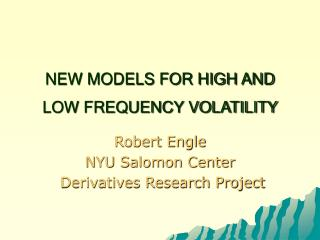DownloadDownload PresentationNEW MODELS FOR HIGH AND LOW FREQUENCY VOLATILITY

# NEW MODELS FOR HIGH AND LOW FREQUENCY VOLATILITY

Télécharger la présentation## NEW MODELS FOR HIGH AND LOW FREQUENCY VOLATILITY

- - - - - - - - - - - - - - - - - - - - - - - - - - - E N D - - - - - - - - - - - - - - - - - - - - - - - - - - -
##### Presentation Transcript

1. NEW MODELS FOR HIGH AND LOW FREQUENCY VOLATILITY Robert Engle NYU Salomon Center Derivatives Research Project

2. FORECASTING WITH GARCH

3. DJ RETURNS

4. DOW JONES SINCE 1990 Dependent Variable: DJRET Method: ML - ARCH (Marquardt) - Normal distribution Date: 01/13/05 Time: 14:30 Sample: 15362 19150 Included observations: 3789 Convergence achieved after 14 iterations Variance backcast: ON GARCH = C(2) + C(3)*RESID(-1)^2 + C(4)*GARCH(-1) Coefficient Std. Error z-Statistic Prob. C 0.000552 0.000135 4.093478 0.0000 Variance Equation C 9.89E-07 1.84E-07 5.380913 0.0000 RESID(-1)^2 0.066409 0.004478 14.82844 0.0000 GARCH(-1) 0.924912 0.005719 161.7365 0.0000 R-squared -0.000370 Mean dependent var 0.000356 Adjusted R-squared -0.001163 S.D. dependent var 0.010194 S.E. of regression 0.010200 Akaike info criterion -6.557778 Sum squared resid 0.393815 Schwarz criterion -6.551191 Log likelihood 12427.71 Durbin-Watson stat 1.985498

5. DEFINITIONS • rt is a mean zero random variable measuring the return on a financial asset • CONDITIONAL VARIANCE • UNCONDITIONAL VARIANCE

6. GARCH(1,1) • The unconditional variance is then

7. GARCH(1,1) • If omega is slowly varying, then • This is a complicated expression to interpret

8. SPLINE GARCH • Instead, use a multiplicative form • Tau is a function of time and exogenous variables

9. UNCONDITIONAL VOLATILTIY • Taking unconditional expectations • Thus we can interpret tau as the unconditional variance.

10. SPLINE • ASSUME UNCONDITIONAL VARIANCE IS AN EXPONENTIAL QUADRATIC SPLINE OF TIME • For K knots equally spaced

11. ESTIMATION • FOR A GIVEN K, USE GAUSSIAN MLE • CHOOSE K TO MINIMIZE BIC FOR K LESS THAN OR EQUAL TO 15

12. EXAMPLES FOR US SP500 • DAILY DATA FROM 1963 THROUGH 2004 • ESTIMATE WITH 1 TO 15 KNOTS • OPTIMAL NUMBER IS 7

13. RESULTS LogL: SPGARCH Method: Maximum Likelihood (Marquardt) Date: 08/04/04 Time: 16:32 Sample: 1 12455 Included observations: 12455 Evaluation order: By observation Convergence achieved after 19 iterations Coefficient Std. Error z-Statistic Prob. C(4) -0.000319 7.52E-05 -4.246643 0.0000 W(1) -1.89E-08 2.59E-08 -0.729423 0.4657 W(2) 2.71E-07 2.88E-08 9.428562 0.0000 W(3) -4.35E-07 3.87E-08 -11.24718 0.0000 W(4) 3.28E-07 5.42E-08 6.058221 0.0000 W(5) -3.98E-07 5.40E-08 -7.377487 0.0000 W(6) 6.00E-07 5.85E-08 10.26339 0.0000 W(7) -8.04E-07 9.93E-08 -8.092208 0.0000 C(5) 1.137277 0.043563 26.10666 0.0000 C(1) 0.089487 0.002418 37.00816 0.0000 C(2) 0.881005 0.004612 191.0245 0.0000 Log likelihood -15733.51 Akaike info criterion 2.528223 Avg. log likelihood -1.263228 Schwarz criterion 2.534785 Number of Coefs. 11 Hannan-Quinn criter. 2.530420

14. ESTIMATION • Volatility is regressed against explanatory variables with observations for countries and years. • Within a country residuals are auto-correlated due to spline smoothing. Hence use SUR. • Volatility responds to global news so there is a time dummy for each year. • Unbalanced panel

15. ONE VARIABLE REGRESSIONS

16. MULTIPLE REGRESSIONS

17. IMPLICATIONS • Unconditional volatility varies over time and can be modeled • Volatility mean reverts to the level of unconditional volatility • Long run volatility forecasts depend upon macroeconomic and financial fundamentals

18. HIGH FREQUENCY VOLATILITY

19. WHERE CAN WE GET IMPROVED ACCURACY? • USING ONLY CLOSING PRICES IGNORES THE PROCESS WITHIN THE DAY. • BUT THERE ARE MANY COMPLICATIONS. HOW CAN WE USE THIS?

20. ONE MONTH OF DAILY RETURNS

21. INTRA-DAILY RETURNS

22. ARE THESE DAYS THE SAME?

23. CAN WE USE THIS INFORMATION TO MEASURE VOLATILITY BETTER? • DAILY HIGH AND LOW • DAILY REALIZED VOLATILITY

24. PARKINSON(1980) • HIGH LOW ESTIMATOR • IF RETURNS ARE CONTINUOUS AND NORMAL WITH CONSTANT VARIANCE,

25. TARCH MODEL WITH RANGE • C 1.07E-06 2.03E-07 5.268049 0.0000 • RESID(-1)^2 -0.100917 0.011398 -8.853549 0.0000 • RESID(-1)^2*(RESID(-1)<0) 0.096744 0.010951 8.834209 0.0000 • GARCH(-1) 0.879976 0.010518 83.65995 0.0000 • RANGE(-1)^2 0.075963 0.008281 9.172690 0.0000 • Adjusted R-squared -0.001360     S.D. dependent var 0.010323 • S.E. of regression 0.010330     Akaike info criterion -6.616277 • Sum squared resid 0.404010     Schwarz criterion -6.606403 • Log likelihood 12550.46     Durbin-Watson stat 2.001541

26. A MULTIPLE INDICATOR MODEL FOR VOLATILITY USING INTRA-DAILY DATARobert F. Engle Giampiero M. Gallo Forthcoming, Journal of Econometrics

27. Absolute returns • Insert asymmetric effects (sign of returns) • Insert other lagged indicators

28. Repeat for daily range, hlt: And for realized daily volatility, dvt :

29. Smallest BIC-based selection